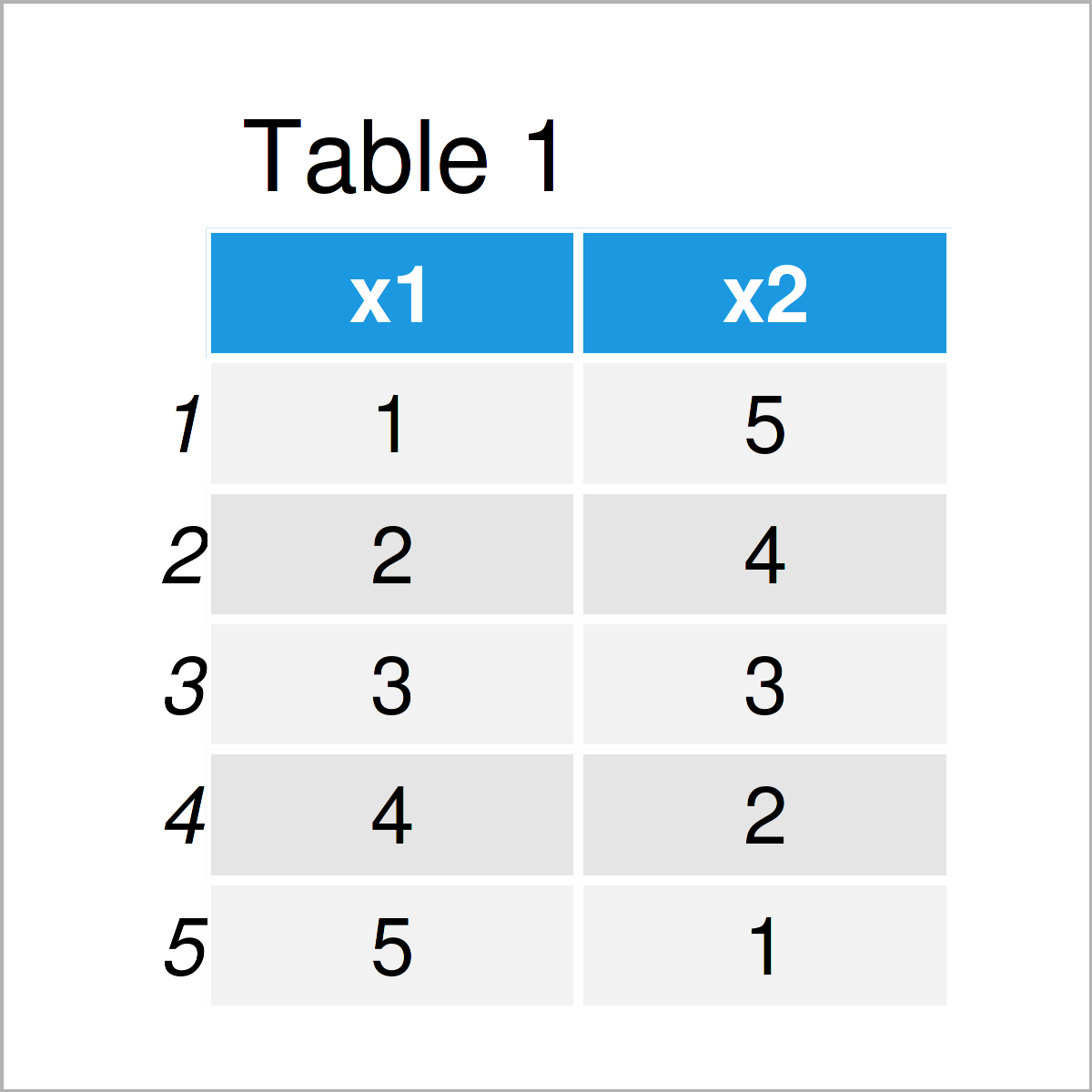# Convert Data Frame to Array in R (Example)

In this article you’ll learn how to save data frames in an array object in R programming.

It’s time to dive into the example:

## Creation of Example Data

The following data is used as a basis for this R programming tutorial:

```data1 <- data.frame(x1 = 1:5,                # Create first data frame
x2 = 5:1)
data1                                        # Print first data frame``````data2 <- data.frame(x1 = 11:15,              # Create second data frame
x2 = 15:11)
data2                                        # Print second data frame```In Tables 1 and 2 it is shown that we have created two data frames containing the same row and column names, but different values.

Let’s put these data frames into an array!

## Example: Save Data Frames in Array Object Using array() Function

This example explains how to create an array based on two input data frames in the R programming language.

For this task, we can use the array, c, unlist, list, rownames, and colnames functions as shown below:

```my_array <- array(data = c(unlist(data1),    # Convert data frames to array
unlist(data2)),
dim = c(5, 2, 2),
dimnames = list(rownames(data1),
colnames(data1)))
my_array                                     # Print array
# , , 1
#
#   x1 x2
# 1  1  5
# 2  2  4
# 3  3  3
# 4  4  2
# 5  5  1
#
# , , 2
#
#   x1 x2
# 1 11 15
# 2 12 14
# 3 13 13
# 4 14 12
# 5 15 11```

As you can see, the previous R syntax has created a new array called my_array that contains our two input data frames.

## Video & Further Resources

Would you like to learn more about the conversion of data frames to an array object? Then I recommend having a look at the following video on my YouTube channel. I show the R programming syntax of this article in the video:

Please accept YouTube cookies to play this video. By accepting you will be accessing content from YouTube, a service provided by an external third party.If you accept this notice, your choice will be saved and the page will refresh.

In addition, you might read some of the other tutorials on this website:

You have learned in this article how to convert, transform, and reshape a data frame to an array in the R programming language. If you have additional questions, don’t hesitate to let me know in the comments.

Subscribe to the Statistics Globe Newsletter